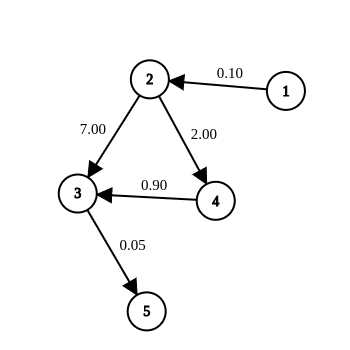# Practice on Toph

Participate in exhilarating programming contests, solve unique algorithm and data structure challenges and be a part of an awesome community.

By reborn · Limits 1s, 256 MBAda is wondering in her dreamland. Her dreamland consists of $n$ spots. She has this weird machine with her that shows real numbers. And whenever she goes from spot $u$ to $v$, if she can (by following a trail), the value shown in the machine gets multiplied with $c_{uv}$ which is another weird value for each trail that exists between two spots.

Ada is currently in spot 1 and her machine currently reads value $d$. She wants to go to spot $n$ in a way so that the machine shows zero when she reaches $n$. Is that possible?

Please note that, Ada can visit a spot multiple times if it helps her.

(Change from printed statement) Ada’s machine displays zero when the value is either zero or tends to be zero. To say that $x$ tends to zero is to say that $x$ varies in such a way that its numerical value becomes and remains less than any positive number that we may choose, no matter how small.

## Input

First line contains two integers and one positive real number $n, ~m, ~d$; the number of spots, the number of trails, the initial reading of the machine respectively.

Each of the next $m$ lines contains two integers $u, ~v$ and a positive real number $c_{uv}$; which corresponds to a trail from spot $u$ to spot $v$ with the multiplier $c_{uv}$.

$c_{uv}$ and $d$ will have exactly 2 digits after the decimal place.

Note that the trails are unidirectional. Also there will be no trail from a spot to itself. And there exists at most one trail from a spot $u$ to $v$.

### Constraints

• $2 \leq n \leq 10^3$.
• $0 \leq m \leq \min (10^5, n(n-1))$.
• $1 \leq u, v \leq n$.
• $0 < c_{uv}, d \leq 10^6$.

## Output

If Ada can not reach spot $n$ anyhow, please output “Unreachable” on a line.
Else, print “Yes” if she can obtain zero at the machine reading after arriving in spot $n$. Print “No” otherwise.

## Sample

InputOutput
5 5 1.00
1 2 0.10
2 3 7.00
2 4 2.00
4 3 0.90
3 5 0.05

NoThere are two ways to go to 5 from 1:
A. 1-2-3-5 yields a value of 1 x 0.10 x 7.00 x 0.05 = 0.035.
B. 1-2-4-3-5 yields a value of 1 x 0.10 x 2.00 x 0.90 x 0.05 = 0.09.

None of them yields 0.

Discussion
Statistics

27% Solution Ratio

NirjhorEarliest, 2w ago

EgorKulikovFastest, 0.2s

NirjhorLightest, 4.1 MB

tmwilliamlin168Shortest, 1142B

Submit